# AP Chemistry : Other Atomic Concepts

## Example Questions

### Example Question #1 : Elements, Ions, And Isotopes

As atomic radius decreases, the force of attraction between the nucleus of the atom and its electrons __________.

cannot be determined

stays the same

increases

decreases

increases

Explanation:

As the atomic radius decreases, electrons are drawn closer to the nucleus. Since the electromagnetic force between the positively charged nucleus and negatively charged electrons is a function of distance, the force of attraction, or effective nuclear charge, exerted on each electron will be greater.

### Example Question #110 : Ap Chemistry

What is the shorthand electron configuration for gallium?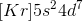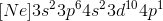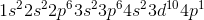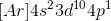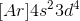Explanation:

To write out the shorthand electronic configuration for Ga, you simply write down the noble gas that that comes before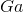, in this case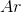, then write the rest of the electron configuration as normal.

### Example Question #1 : Other Atomic Concepts

An electron falling to a lower energy level gives off a blue glow (λ=475nm). How much energy is emitted?

4.184 x 10-17 J

3.14x10-40J

Energy is not emitted

3.14x10-37J

4.184 x 10-19 J

4.184 x 10-19 J

Explanation:

E=hf, or E=hc/λ

h is Planck's constant with a value of 6.62606957 × 10-34 m2 kg / s. Converting 475nm to m gives 4.75x10-7m. c is the speed of light with 3x108m/s. Putting these variables into the second equation you get 4.174x10-19J

### Example Question #2 : Other Atomic Concepts

The atomic number of an atom is equal to the number of __________.

protons

electrons

neutrons

protons + neutrons

electrons + neutrons